Test : Angles And Their Measurement - 2

# Test : Angles And Their Measurement - 2

Test Description

## 10 Questions MCQ Test Mathematics for Class 5: NCERT | Test : Angles And Their Measurement - 2

Test : Angles And Their Measurement - 2 for Class 5 2023 is part of Mathematics for Class 5: NCERT preparation. The Test : Angles And Their Measurement - 2 questions and answers have been prepared according to the Class 5 exam syllabus.The Test : Angles And Their Measurement - 2 MCQs are made for Class 5 2023 Exam. Find important definitions, questions, notes, meanings, examples, exercises, MCQs and online tests for Test : Angles And Their Measurement - 2 below.
Solutions of Test : Angles And Their Measurement - 2 questions in English are available as part of our Mathematics for Class 5: NCERT for Class 5 & Test : Angles And Their Measurement - 2 solutions in Hindi for Mathematics for Class 5: NCERT course. Download more important topics, notes, lectures and mock test series for Class 5 Exam by signing up for free. Attempt Test : Angles And Their Measurement - 2 | 10 questions in 10 minutes | Mock test for Class 5 preparation | Free important questions MCQ to study Mathematics for Class 5: NCERT for Class 5 Exam | Download free PDF with solutions
 1 Crore+ students have signed up on EduRev. Have you?
Test : Angles And Their Measurement - 2 - Question 1

### ​Danish drew an angle as shown here. Manish drew an angle that was twice the measure of Danish's angle. What was the measure of Manish's angle?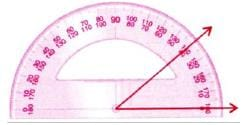Detailed Solution for Test : Angles And Their Measurement - 2 - Question 1

Measure of angle drawn by Danish = 40°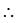Measure of angle drawn by Manish = 2 x 40° = 80°

Test : Angles And Their Measurement - 2 - Question 2

### At 3:25, the smaller angle formed between the two hands of a clock is ____.

Detailed Solution for Test : Angles And Their Measurement - 2 - Question 2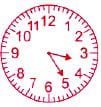Test : Angles And Their Measurement - 2 - Question 3

### ​How many of the given figures have perpendicular lines?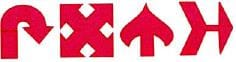Test : Angles And Their Measurement - 2 - Question 4

​Which type of angle best describes angle Q?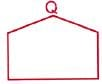Test : Angles And Their Measurement - 2 - Question 5

​How many angles are less than 90° in the given figures?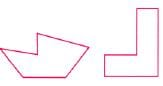Test : Angles And Their Measurement - 2 - Question 6

There are six angles below. How many of them are greater than a right angle?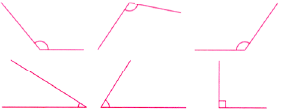Test : Angles And Their Measurement - 2 - Question 7

​In the given figure, which line is perpendicular to AB?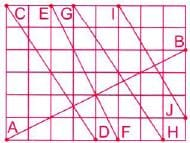Detailed Solution for Test : Angles And Their Measurement - 2 - Question 7

EF is perpendicular to AB

Test : Angles And Their Measurement - 2 - Question 8

Which of the following is greater than a right angle?

Test : Angles And Their Measurement - 2 - Question 9

​What is the approximate measure of this angle in degrees?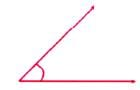Detailed Solution for Test : Angles And Their Measurement - 2 - Question 9

The given angle is a acute angle.
The approximate value of above angle is 45°
, as the angle of 20°  would be very small than the given angle Hence the correct answer is option B.

Test : Angles And Their Measurement - 2 - Question 10

An angle which measures more than 90° but less than 180° is called_____.

Detailed Solution for Test : Angles And Their Measurement - 2 - Question 10

An angle between 90 and 180 degree is called an obtuse angle.

## Mathematics for Class 5: NCERT

36 videos|73 docs|45 tests
 Use Code STAYHOME200 and get INR 200 additional OFF Use Coupon Code
Information about Test : Angles And Their Measurement - 2 Page
In this test you can find the Exam questions for Test : Angles And Their Measurement - 2 solved & explained in the simplest way possible. Besides giving Questions and answers for Test : Angles And Their Measurement - 2, EduRev gives you an ample number of Online tests for practice

## Mathematics for Class 5: NCERT

36 videos|73 docs|45 tests